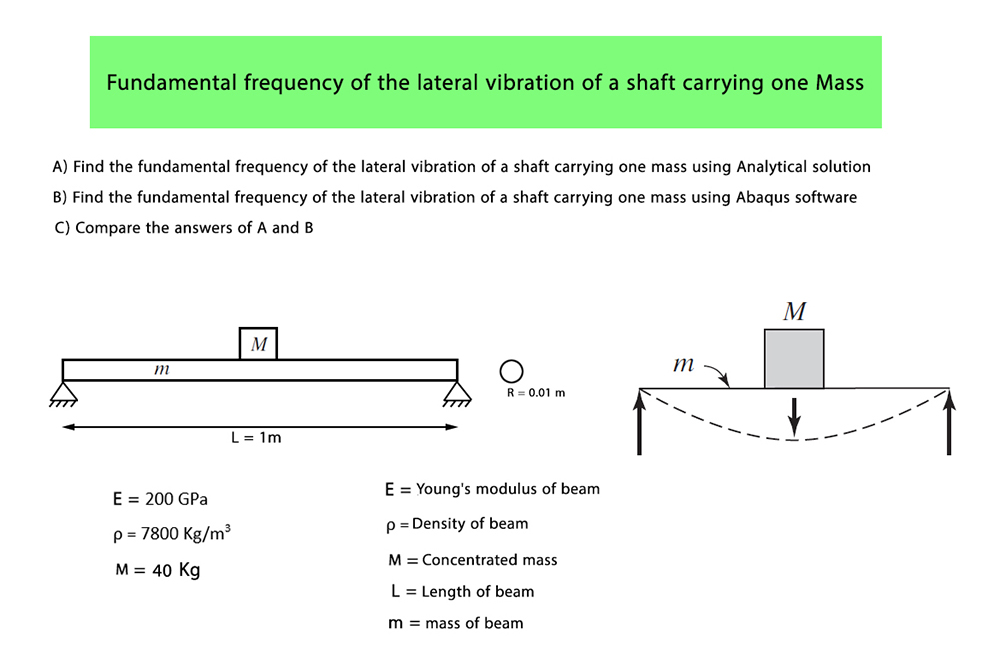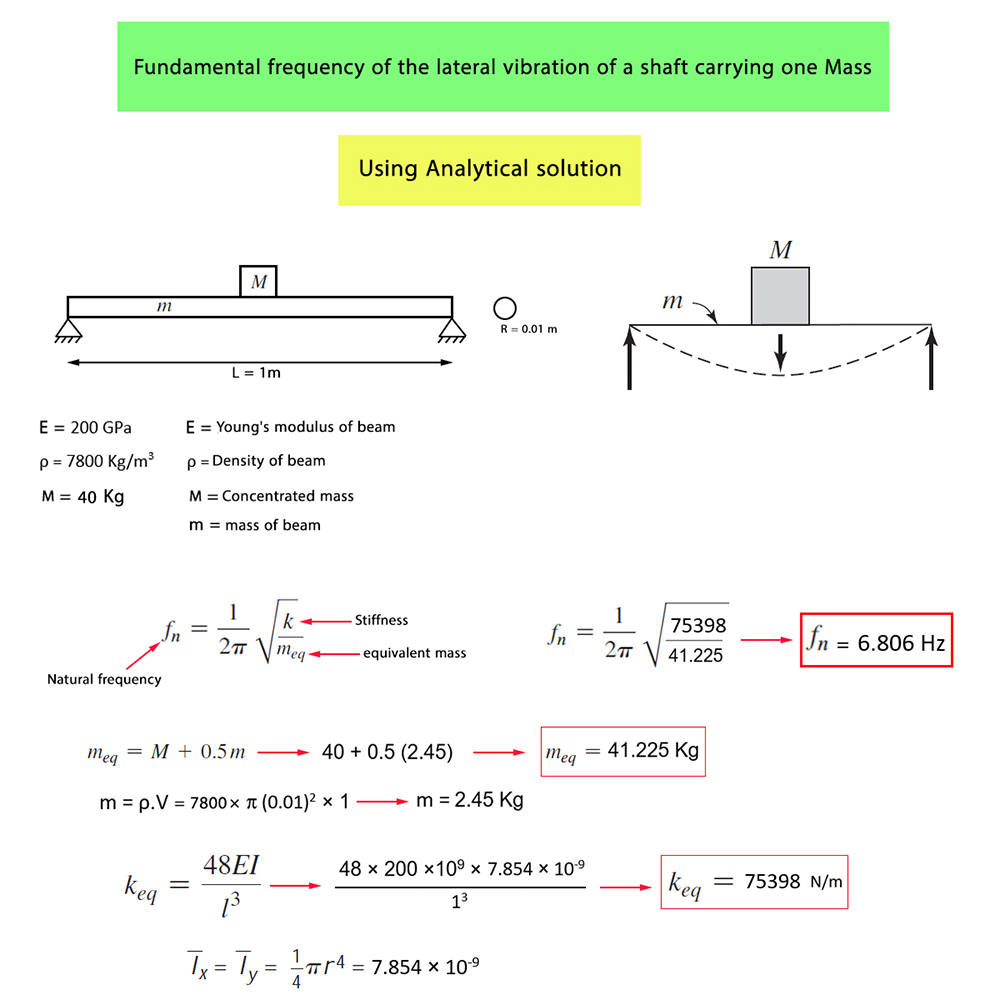Sale!

0

Fundamental Frequency of the Lateral Vibration of a Shaft carrying one mass using the Abaqus software and Analytical Solution

This tutorial is free

## Description

Fundamental Frequency of the Lateral Vibration of a Shaft carrying one mass using the Abaqus software and Analytical Solution

In this illustration, we intend to calculate the Fundamental Frequency of the Lateral Vibration of a Shaft carrying one mass using the Abaqus software and Analytical Solution

As you observe in the picture, the Model includes Beam and a Concentrated Mass which is located exactly on the center of the beam

The beam is one meter in length and the cross-section of the beam is a circle with a radius equal to 0.01 m. Meanwhile, a hinged support has been used on both sides of the beamThe purpose of this training is to calculate the fundamental frequency of the lateral vibration of the shaft with concentrated Mass using the Abacus software and then compare the results obtained from the Abacus software with those gained from the Analytical Solution. Actually, this problem will be solved twice, once using the Analytical Solution and for the second time, by using the Abacus softwareThe natural frequency having been obtained from the Analytical Solution is 6.806 HertzThe natural frequency having been obtained from the Abaqus software is equal to 6.806 Hertz

As you observe, the results obtained from the Analytical Solution are exactly concordant with those gained from the Abaqus software